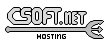<-- Back to AG_Intro.3

# SYNOPSIS

 ```#include #include #include ```

# DESCRIPTION

The M_Plane structure describes a plane in R3 (Ax+By+Cz+D=0):
 ```typedef struct m_plane { M_Vector3 n; /* Normal vector */ M_Real d; /* Distance to origin */ } M_Plane; ```

The plane satisfies the equation A(n.x) + B(n.y) + C(n.z) = d.

# INITIALIZATION

 M_Plane M_PlaneFromPts (M_Vector3 p1, M_Vector p2, M_Vector p3)M_Plane M_PlaneFromParallel (M_Plane P, M_Real d)M_Plane M_PlaneRead (AG_DataSource *ds)void M_PlaneWrite (AG_DataSource *ds, M_Plane *P)M_Plane M_PLANE_INITIALIZER (M_Real a, M_Real b, M_Real c, M_Real d)

The M_PlaneFromPts() function returns a plane from three (non-colinear) points p1, p2 and p3. M_PlaneFromParallel() returns the parallel plane at distance d from the specified plane P.

The M_PlaneRead() and M_PlaneWrite() functions read or write a plane structure from/to an AG_DataSource(3).

The macro M_PLANE_INITIALIZER() expands to a static initializer for a M_Plane from the coefficients of the plane equation a, b, c, d.

# TESTS

 M_Real M_PlaneVectorAngle (M_Plane P, M_Vector3 v)

The M_PlaneVectorAngle() function computes the angle between the plane and specified vector, in radians.